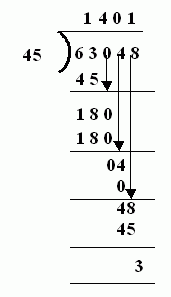Name: ___________________Date:___________________

 Email us to get an instant 20% discount on highly effective K-12 Math & English kwizNET Programs!

### MEAP Preparation - Grade 6 Mathematics2.40 Division of Large Numbers - Two Digit Divisor

 Look at the first two numbers of the dividend, if this is lesser than the divisor, consider the next digit too. Divide these digits by the divisor, subtract and then bring down the next digit of the dividend. Continue the same steps till the dividend is completely divided by the divisor. Example:Answer: 1401R3 Directions: Divide the following. Also write at least five examples of your own.
 Question 1: 403746 / 21

Question 2: This question is available to subscribers only!

Question 3: This question is available to subscribers only!

Question 4: This question is available to subscribers only!

Question 5: This question is available to subscribers only!

Question 6: This question is available to subscribers only!